# Introduction to Deep Learning¶

### First import necessary helper libraries for this tutorial and define helper functions¶

In :
import numpy as np
from pylab import *
%matplotlib inline

# You'll also need:
# $pip install mxnet #$pip install xfer-ml

In :
# Define helper function for later

def plot_point(func, t, xmin, xmax):
N = 10
x = np.zeros((N,1))+t
x = x[:,0]
y = np.linspace(0,func(t),N)
plt.plot(x,y, 'k--', linewidth=1)

x = np.linspace(xmin, t, N)
y = np.zeros((N,1))+func(t)
plt.plot(x,y, 'k--', linewidth=1)


### Define the activation function and its derivative¶

In :
# Sigmoid activation function
def activation(x):
return 1/(1+np.exp(-x))

# Derivative of activation wrt input x
def activation_derivative(x):
# the activation of the sigmoid function conveniently can be written in terms of its outputs.
f = activation(x)
return f*(1-f)

# Derivative of activation wrt input x but expressed when activation(x) is given as argument instead of x
def activation_derivative_f(f):
return f*(1-f)


### Plots to explore the activation¶

First, plot the activation for input domain between -10 and 10

In :
x1 = np.linspace(-10, 10, 500)
plt.plot(x1, activation(x1))
plt.xlabel('x'); plt.ylabel('activation(x)')

Out:
Text(0,0.5,'activation(x)')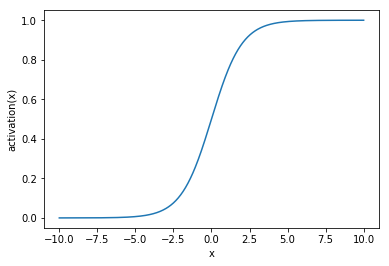Let's see what happens with low, intermediate and high values:

In :
x1 = np.linspace(-10, 10, 500)
plt.plot(x1, activation(x1))
plt.xlabel('x'); plt.ylabel('activation(x)')
plot_point(activation, x1, -10, 10)
plot_point(activation, x1, -10, 10)
plot_point(activation, x1, -10, 10)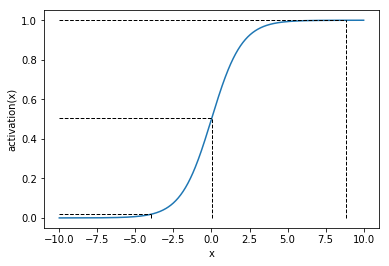Repeat but for the space -20 to 20

In :
x2 = np.linspace(-20, 20, 500)
plt.plot(x2, activation(x2))
plt.xlabel('x'); plt.ylabel('activation(x)')
plot_point(activation, x1, -20, 20)
plot_point(activation, x1, -20, 20)
plot_point(activation, x1, -20, 20)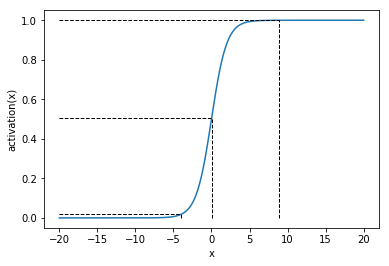Explore the derivative

In :
plt.plot(x1, activation(x1))
plt.plot(x1, 4*activation_derivative(x1), '--')
plt.plot(np.zeros((5,1)), np.linspace(0,1,5), '--')
plt.legend(['activation','activation_derivative','decision boundary'])
plt.xlabel('x')
plt.ylabel('activation(x)')

Out:
Text(0,0.5,'activation(x)')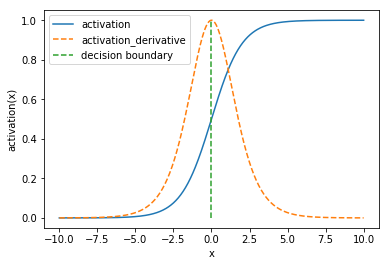## Logistic regression¶

In :
# Create some toy data
X = np.array([[0,0,1],
[0,1,1],
[1,0,1],
[1,1,1]])

# Linear case
y = np.array([,
,
,
])

# Fix the seed
np.random.seed(1)

In :
# Initialize weight
W0 = 2*np.random.random((3,1)) - 1
error = []
error_of_rounded = []
fs = []

# Optimize for 2000 iterations
for i in range(200):
# FORWARD PASS: x -> f1
f0 = X
f1 = activation(np.dot(f0, W0))
loss = (0.5 * (y-f1)**2 ).sum()
loss_of_rounded = (0.5 * (y-np.round(f1))**2 ).sum()

# BACKWARDS PASS (derivatives of loss wrt W0)
e1 = y - f1   # Error contribution
delta_1 = e1 * activation_derivative_f(f1)  # The gradient contribution from activation

learning_rate = 0.2

# Update the weights. This is simply adding the gradient multiplied by a learning rate

# Keep track of error
error.append(loss)
error_of_rounded.append(loss_of_rounded)
fs.append(f1)

plt.plot(error)
plt.xlabel('number of iterations')
plt.ylabel('error in training data')

plt.figure()
plt.plot(error_of_rounded)
plt.xlabel('number of iterations')
plt.ylabel('error in training data')

Out:
Text(0,0.5,'error in training data')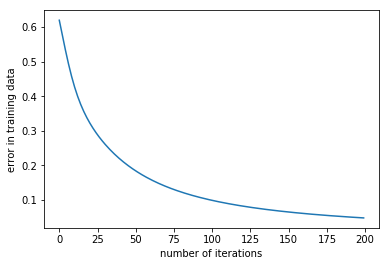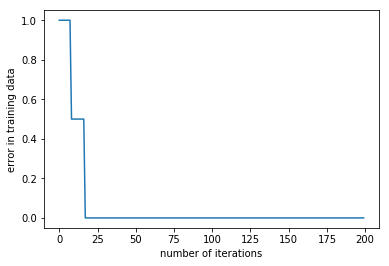## Deep neural network¶

Create some toy data

In :
X = np.array([[0,0,1],
[0,1,1],
[1,0,1],
[1,1,1]])

# NON-linear case
y = np.array([,
,
,
])


Define parameters and initialize them.

In :
# Fix the seed
np.random.seed(1)

# randomly initialize our weights with mean 0
W0 = 2*np.random.random((3,4)) - 1
W1 = 2*np.random.random((4,1)) - 1


Optimize!

In :
error = []
error_of_rounded = []

# Optimize
for i in range(1000):
# FORWARD PASS: x -> f1 -> f2
f0 = X
f1 = activation(np.dot(f0, W0))
f2 = activation(np.dot(f1, W1))
# Normally we'd use the cross-entropy loss, but here we'll use sq. error to make derivatives easier.
loss = (0.5 * (y-f2)**2 ).sum()
loss_of_rounded = (0.5 * (y-np.round(f2))**2 ).sum()

# BACKWARDS PASS
e2 = y - f2
delta_2 = e2 * activation_derivative_f(f2)

e1 = np.dot(delta_2, W1.T)
delta_1 = e1 * activation_derivative_f(f1)

# Update the weights
learning_rate = 0.8

# Keep track of error
error.append(loss)
error_of_rounded.append(loss_of_rounded)


Plot the error per iteration of the optimization.

In :
plt.plot(error)
plt.xlabel('number of iterations')
plt.ylabel('error in training data')

plt.figure()
plt.plot(error_of_rounded)
plt.xlabel('number of iterations')
plt.ylabel('error in training data')

Out:
Text(0,0.5,'error in training data')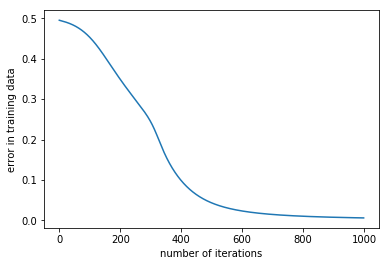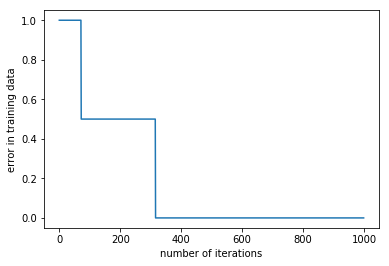### With Automatic differentiation¶

In :
from __future__ import print_function
import mxnet as mx
from mxnet import nd, autograd, gluon

np.random.seed(1)
mx.random.seed(1)


Define the parameters.

In :
W0 = 2*nd.random.randn(3,4, ctx=mx.cpu()) - 1
W1 = 2*nd.random.randn(4,1, ctx=mx.cpu()) - 1
params = [W0, W1]
for param in params:


Define the neural network structure.

In :
def activation_mx(x):
return 1/(1+nd.exp(-x))

def net(X):
f1 = activation_mx(nd.dot(X, W0))
f2 = activation_mx(nd.dot(f1, W1))
return f2


Do optimization!

In :
error = []
error_of_rounded = []

for i in range(2000):
# We do forward pass while recording the symbolic graph so as to be able to do automatic differentiation
# FORWARD PASS
f2 = net(nd.array(X))
loss = nd.sum(0.5 * (nd.array(y)-f2)**2 )
loss_of_rounded = nd.sum(0.5 * (nd.array(y)-np.round(f2))**2 )

# BACKWARDS PASS
loss.backward()

# PARAMETER UPDATE
for param in params:
param[:] = param - 0.8 * param.grad

# Keep track of error
error.append(loss.asscalar())
error_of_rounded.append(loss_of_rounded.asscalar())



Plot the error per iteration of the optimization:

In :
plt.plot(error)
plt.xlabel('number of iterations')
plt.ylabel('error in training data')

plt.figure()
plt.plot(error_of_rounded)
plt.xlabel('number of iterations')
plt.ylabel('error in training data')

Out:
Text(0,0.5,'error in training data')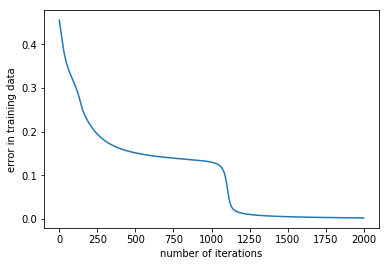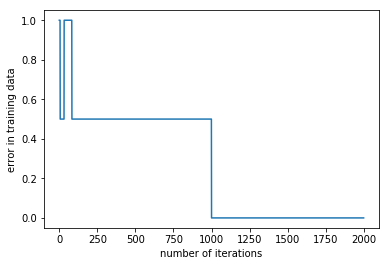## Reusing deep neural networks¶

In :
import xfer
# You can install it with:
#    \$pip install xfer-ml

# Or clone and install from souce:
#    https://github.com/amzn/xfer.git

In :
path = 'http://data.mxnet.io/models/imagenet/'

Out:
['squeezenet_v1.1-0000.params', 'squeezenet_v1.1-symbol.json']

Just utility functions to handle data.

In :
import json
def get_iterators_from_folder(data_dir, train_size=0.6, batchsize=10, label_name='softmax_label', data_name='data', random_state=1):
import os,glob
from sklearn.model_selection import StratifiedShuffleSplit
"""
Method to create iterators from data stored in a folder with the following structure:
/data_dir
/class1
class1_img1
class1_img2
...
class1_imgN
/class2
class2_img1
class2_img2
...
class2_imgN
...
/classN
"""
# assert dir exists
if not os.path.isdir(data_dir):
# get class names
classes = [x.split('/')[-1] for x in glob.glob(data_dir+'/*')]
classes.sort()
fnames = []
labels = []
for c in classes:
# get all the image filenames and labels
images = glob.glob(data_dir+'/'+c+'/*')
images.sort()
fnames += images
labels += [c]*len(images)
# create label2id mapping
id2label = dict(enumerate(set(labels)))
label2id = dict((v,k) for k, v in id2label.items())

# get indices of train and test
sss = StratifiedShuffleSplit(n_splits=2, test_size=None, train_size=train_size, random_state=random_state)
train_indices, test_indices = next(sss.split(labels, labels))

train_img_list = []
test_img_list = []
train_labels = []
test_labels = []
# create imglist for training and test
for idx in train_indices:
train_img_list.append([label2id[labels[idx]], fnames[idx]])
train_labels.append(label2id[labels[idx]])
for idx in test_indices:
test_img_list.append([label2id[labels[idx]], fnames[idx]])
test_labels.append(label2id[labels[idx]])

# make iterators
train_iterator = mx.image.ImageIter(batchsize, (3,224,224), imglist=train_img_list, label_name=label_name, data_name=data_name,
path_root='')
test_iterator = mx.image.ImageIter(batchsize, (3,224,224), imglist=test_img_list, label_name=label_name, data_name=data_name,
path_root='')

return train_iterator, test_iterator, train_labels, test_labels, id2label, label2id

def get_images(iterator):
"""
Returns list of image arrays from iterator
"""
iterator.reset()
images = []
while True:
try:
batch = iterator.next().data
for n in range(batch.shape):
images.append(batch[n])
except StopIteration:
break
return images

def show_predictions(predictions, images, id2label, uncertainty=None, figsize=(9,1.2), fontsize=12, n=8):
"""
Plots images with predictions as labels. If uncertainty is given then this is plotted below as a
series of horizontalbar charts.
"""
num_rows = 1 if uncertainty is None else 2

plt.figure(figsize=figsize)
for cc in range(n):
plt.subplot(num_rows,n,1+cc)
plt.tick_params(
axis='both',          # changes apply to the x-axis
which='both',      # both major and minor ticks are affected
bottom=False,      # ticks along the bottom edge are off
top=False,         # ticks along the top edge are off
left=False,
labelleft=False,
labelbottom=False) # labels along the bottom edge are off
plt.imshow(np.uint8(images[cc].asnumpy().transpose((1,2,0))))
plt.title(id2label[predictions[cc]].split(','), fontsize=fontsize)
plt.axis

# This utility just allows us to translate image-id's of the imagenet dataset to human-readable labels
with open('imagenet1000-class-to-human.json', 'r') as fp:

imagenet_class_to_human = {int(k): v for k, v in imagenet_class_to_human.items()}

In :
# Load train and test data
train_iterator,test_iterator, train_labels, test_labels, id2label, label2id = get_iterators_from_folder('test_sketches', 0.6, 4, label_name='prob_label', random_state=1)


In :
source_model = mx.module.Module.load('squeezenet_v1.1', 0, label_names=['prob_label'])
mh = xfer.model_handler.ModelHandler(source_model) # Attach the source model to xfer


Let's try to predict with the pre-trained network.

In :
# Get pre-trained model without modifications
model = mh.get_module(iterator=test_iterator)
# Predict on our test data
predictions = np.argmax(model.predict(test_iterator), axis=1).asnumpy().astype(int)

# Plot all test images along with the predicted labels
images = get_images(test_iterator)
show_predictions(predictions, images, imagenet_class_to_human, None, (15, 1.5))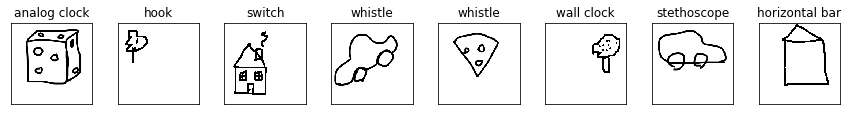That clearly doesn't work. The images we have are different in nature that the ones that the pre-trained (source) model was trained on. Furthermore, the pre-trained images of the source model might not contain some of the labels we have here at all.

So far, we have agreed that: (a) Training a neural network from scratch won't work in this case, since we have very few data. (b) Re-using a pre-trained neural netwrok as is doesn't work either.

Solution is Repurposing: take a pre-trained neural network and repurpose it for the new task through transfer learning.

In :
repLR = xfer.LrRepurposer(source_model=source_model, feature_layer_names=['pool10'])
repLR.repurpose(train_iterator)
predictionsLR = repLR.predict_label(test_iterator)

/Users/damianou/anaconda/lib/python3.5/site-packages/sklearn/linear_model/sag.py:334: ConvergenceWarning: The max_iter was reached which means the coef_ did not converge
"the coef_ did not converge", ConvergenceWarning)

In :
show_predictions(predictionsLR, images, id2label, None, (15,1.5))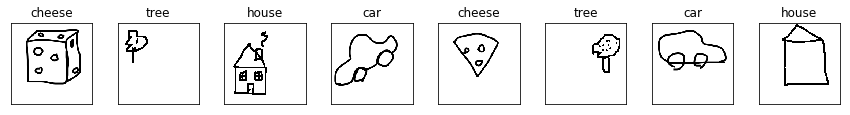Much better!

Other utilities: refining existing architectures really easily!

In :
print("Bottom layer name before addition: " + mh.layer_names)
conv1 = mx.sym.Convolution(name='new_conv_layer', kernel=(20,20), num_filter=64)
print("Bottom layer name after addition: " + mh.layer_names)

# Dropping layers is also supported. The commented code below drops two top layers
# mh.drop_layer_top(2)

conv1
new_conv_layer

In :
from IPython.display import HTML
HTML('<img src="local_images/xfer_metamodel.gif">')

In :
HTML('<img src="local_images/xfer_modelhandler.gif">')